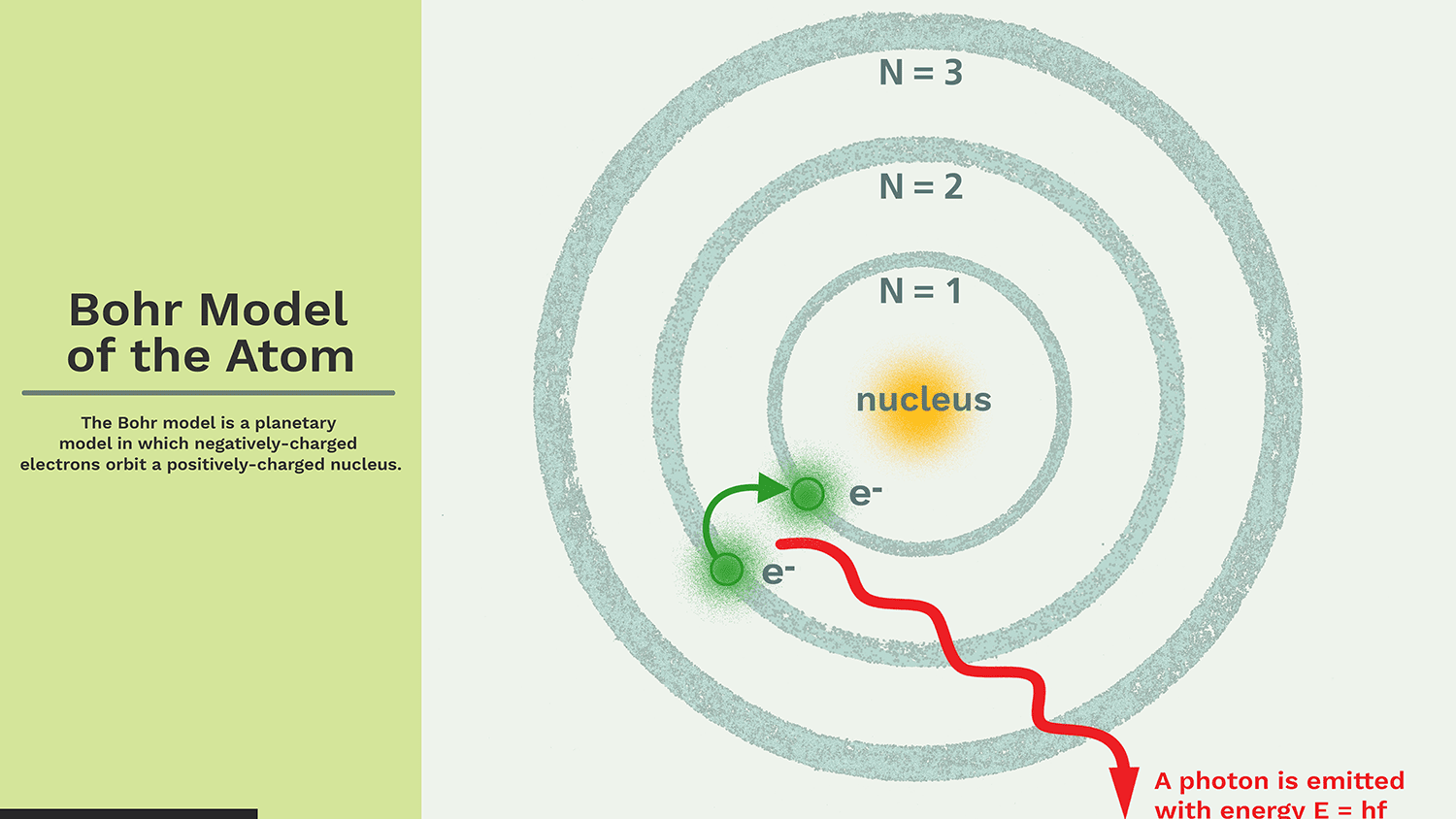# Bohr’s Atomic Model Study Guide

INTRODUCTION

This is a space model in which negatively charged electrons orbit a small positively charged nucleus, much like a planet orbits the Sun (unless the orbit is non-planar). The attractive force of the solar system is mathematically equivalent to the Coulomb force (electric force) that exists between a positively charged nucleus and a negatively charged electron.

## THE BOHR MODEL’S KEY POINTS

• It orbits around the core in a fixed size, fixed energy orbit.

• The energy of the orbit is proportional to its size. The lowest energy is in the smallest orbit.

• Whenever an electron transitions from one orbit to another, it absorbs or emits radiation.

## POSTULATES OF BOHR’S ATOMIC MODEL

• Electrons in an atom (negatively charged) rotate in a specific circular path around a positively charged nucleus.

• These circular orbits are called orbital shells because each orbital or shell has defined energy.

• Quantum numbers are integers (n = 1, 2, 3, …) that represent energy levels. This quantum number range starts from the core side and has the lowest energy level of n = 1. Orbit n = 1, 2, 3, 4 … K, L, M, N …. are assigned to the shell and are considered to be in the ground state when the electron reaches the lowest energy level.

• The electrons in an atom gain energy and move from a low energy level to a high energy level, and the electrons lose energy and move from a high energy level to a low energy level.Source

## LIMITATIONS OF BOHR’S ATOMIC MODEL

• The Bohr model gives an inaccurate value to the ground state orbital angular momentum.
• It has a terrible track record of predicting the spectrum of larger atoms.
• It violates Heisenberg’s Uncertainty Principle because it assumes that the electron has both a known radius and orbit.
• The relative intensity of the spectral lines is unpredictable.
• The microstructure and hyperfine structure of the spectral lines are not explained in the Bohr model.
• It does not exist in the Zeeman effect.

## BOHR’S THEORY IS APPLICABLE TO:

Only single electron species are covered by Bohr’s hypothesis.

It’s a system made up of a compact, dense nucleus surrounded by orbiting electrons, similar to the Solar System, but with electrostatic forces acting as the attraction instead of gravity.

Note – It’s worth noting that Bohr’s atomic model isn’t the most accurate atomic model currently available.

# SUMMARY

• The atomic model was proposed by Niels Bohr.
• Electrons in the Bohr model of the atom travel in circular orbits around the nucleus.
• An integer, the quantum number n, is used to designate the orbits.
• By generating or absorbing energy, electrons can leap from one orbit to the next.

## FAQs

Q. What is Bohr’s Model of an Atom?

In Bohr’s concept, a small (positively charged) nucleus is surrounded by negative electrons that move in orbit around the nucleus. Bohr found that electrons far from the core have more energy than electrons near the core.

Q. How do electrons move in Bohr’s model?

Electrons in Bohr atomic model travel in prescribed circular orbits around the nucleus.

Q. How did Bohr discover electrons?

Niels Bohr offered a theory for the hydrogen atom in 1913, based on quantum theory, which states that some physical quantities have only discrete values.

Q. Did Bohr’s model have neutrons?

The atom is depicted as an atomic nucleus having protons and neutrons, with electrons in circular orbitals at particular distances from the nucleus, according to the Bohr model.

We hope you enjoyed studying this lesson and learned something cool about Bohr’s Atomic Model! Join our Discord community to get any questions you may have answered and to engage with other students just like you! We promise, it makes studying much more fun!😎

## REFERENCE

1. Bohr’s Model of an Atom: https://byjus.com/chemistry/bohrs-model/Accessed 19th April 2022.
2. Bohr Model of Atom: https://www.thoughtco.com/bohr-model-of-the-atom-603815 Accessed 19th April 2022.
3. Bohr’s Atomic Model: https://www.expii.com/t/bohrs-atomic-model-overview-importance-11059 Accessed 19th April 2022.
]]>